# Introduction to distributed systems

What is a distributed system?

According to Wikipedia: A distributed system is a software system in which components located on networked computers communicate and coordinate their actions by passing messages.

## Parallel and distributed systems

• Parallel systems use shared memory
• Distributed parallel systems still use the notion of shared memory, but this is co-ordinated with special HW/SW that unifies memory accesses accross multiple computers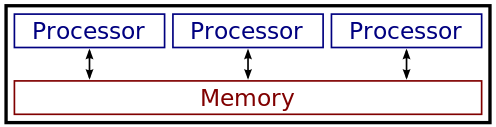Parallel system

• Distributed systems use no shared components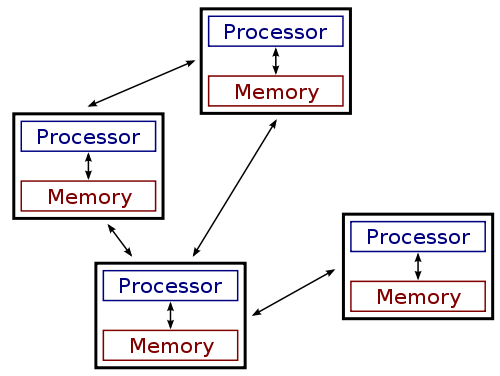Distributed system

## Why distributed systems?

• Scalability
• Moore’s law: The number of transistors on a single chip doubles about every two year.
• The advancement has slowed since around 2010.
• Distribution provides massive performance.
• Distribution of tasks and collaboration
• Reduced Latency
• Fault tolerance
• Mobility

## Distributed system characteristics

• Computational entities each with own memory
• Need to synchronize distributed state
• Entities communicate with message passing
• Each entity maintains parts of the complete picture
• Need to tolerate failure

## Building distributed systems is hard

• They fail often (and failure is difficult to spot!)
• Split-brain scenarios
• Maintaining order/consistency is hard
• Coordination is hard
• Partial operation must be possible
• Testing is hard
• Profiling is hard: “it’s slow” might be due to 1000s of factors

## Fallacies of distributed systems

• The network is reliable
• Latency is zero
• Bandwidth is infinite
• The network is secure
• Topology does not change
• Transport cost is zero
• The network is homogeneous

## Five main problems

We will focus on the following four issues with distributed systems

• Partial failures: Some parts of the system may fail nondeterministically, while other parts work fine.
• Unreliable networks: Distributed systems communicate over unreliable networks.
• Unreliable time: Time is a universal coordination principle. However, we cannot use time determine order.
• No single source of truth: Distributed systems need to co-ordinate and agree upon a (version of) truth.
• Different opinions: Distributed systems need to provide guarantees for consistency in query answers.

# Partial failures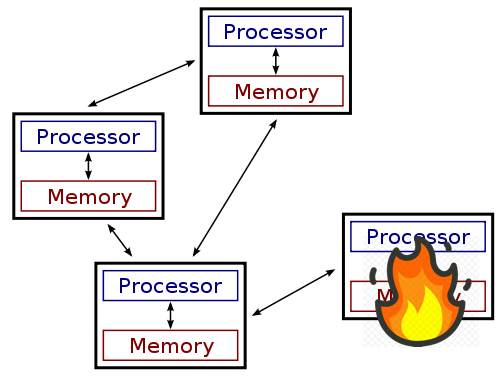Partial failures

Distributed systems must tolerate partial failures: Any one point in the system can fail, yet the system must continue to correctly function as a whole.

Partial failures occur frequently in large distributed systems and they may cause real-world outages.

Hard to detect whether something failed or not, as the time it takes for a message to travel across a network.

# Unreliable networks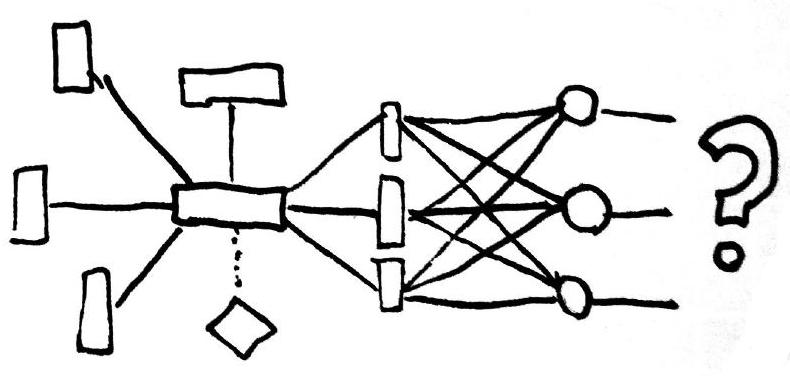Unreliable networks

## How can a network fail?

Q: Imagine a client server application. The client sents a message to the server, but receives no response. What might have gone wrong?

A: Has the service failed? Is the request or response message lost in the network?

## Asynchronous vs synchronous Systems

Two types of network systems:

• Synchronous system: Process execution speeds or message delivery times are bounded.

• Asynchronous system: No assumptions about process execution speeds or message delivery times are made.

Purely synchronous systems only exist in theory.

Most distributed systems use some form of asynchronous networking to communicate.

## Failures in asynchronous systems

Upon waiting for a response to a requests in an asynchronous system, it is not possible to distinguish whether:

1. the request was lost
2. the remote node is down
3. the response was lost

The usual remedy is to set timeouts and retry the request until it succeeds.

## Network failures in practice

In a study of network failures by Microsoft Research, they found that:

• 5 devices per day fail
• 41 links per day fail
• Load balancers fail with a probability >20% once per year
• MMTR 5 mins
• Redundancy is not very effective
• Most failures are due to misconfiguration

The data is for a professionally managed data centre by a single company.

On the public cloud, failures may affect thousands of systems in parallel.

## Timeouts

Timeouts is a fundamental design choice in asynchronous networks: Ethernet, TCP and most application protocols work with timeouts.

The problem with timeouts is that delays in asynchronous systems are unbounded. This can be due to:

• Queueing of packets at the network level, due to high or spiking traffic
• Queueing of requests at the application level, e.g. because the application is busy processing other requests

Queues also experience a snowball effect; queues are getting bigger on busy systems.

Timeouts usually follow the exponential back-off rule; we double the time we check for an answer up to an upper bound. More fine-grained approaches use successful request response times to calibrate appropriate timeouts.

# Unreliable timeSoft Watch At The Moment Of First Explosion, by Salvador Dali

## Time is essential

In a distributed system, time is the only global constant nodes can rely on to make distributed decisions on ordering problems.

When do we need to order?

• Sequencing items in memory (e.g., with a mutex)
• Encoding history (“happens before” relationships)
• Transactions in a database
• Debugging (finding the root cause of a bug)

## Time in computer systems

Time in computers is kept in two ways:

• “Real” time clocks (RTCs): Capture our intuition about time and are kept in sync with the NTP protocol with centralised servers. (e.g. System.getCurrentTimeMillis).
• Monotonic clocks: Those clocks only move forward. (e.g. System.nanoTime)

Q: Which clock type would you use to benchmark a routine? Why?

## The trouble with computer clocks

Monotonic clocks are maintained by the OS and rely on HW counters exposed by CPUs. They are (usually!) good for determining order within a node, but each node only has its own notion of time.

NTP can synchronize time across nodes with an accuracy of ms. A modern CPU can execute $$10^6$$ instructions ($$\times$$ number of cores) in an ms!

Moreover, leap seconds are introduced every now and then; minutes may last for 61 or 59 seconds on occasion

$$\mu s$$ accuracy is possible with GPS clocks, but expensive

## Logical Time

Logical time abstracts the notion of time and orders events based on causality.

If some event possibly causes another event, then the first event happened-before the other.

Lamport introduced the eponymous logical timestamps in 1978 to capture happened-before relation.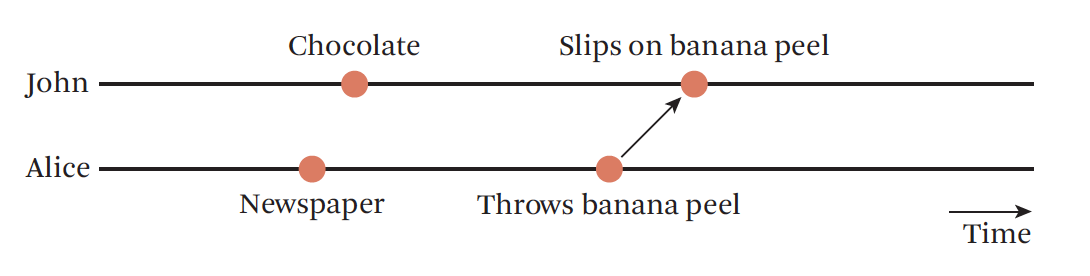Logical time

## Order

What is order? A way of arranging items in a set so that the following properties are maintained.

Strict partial order:

• Irreflexivity: $$\forall a. \neg a < a$$ (items not comparable with self)
• Transitivity: if $$a \le b$$ and $$b \le c$$ then $$a \le c$$
• Antisymmetry: if $$a \le b$$ and $$b \le a$$ $$a = b$$

Strict total order:

• An additional property: $$\forall a, b, a \le b \vee b \le a \vee a = b$$

When do we need to order?

• Sequencing items in memory (e.g. with a mutex)
• Encoding history (“happens before” relationships)
• Transactions in a database

## Order and time

• FIFO is enough to maintain order with a single sender
• Time at the receiver end is enough to maintain order at the receiver end
• When multiple senders/receivers are involved, we need external ordering scheme
• Total order: If our message rate is globally bounded (e.g. 1 msg/sec/receiver), and less fine-grained than our clock accuracy (e.g. ms range), then synchronized RTCs are enough to guarantee order.
• Causal order: Otherwise, we need to rely on happens before ($$\rightarrow$$) relationships.

## Happens-before relation

Lamport introduced happens-before relation to capture dependencies between events:

• If $$a$$ and $$b$$ are events in the same node, and $$a$$ occurs before $$b$$, then $$a \rightarrow b$$.
• If $$a$$ is the event of sending a message and $$b$$ is the event of receiving that message, then $$a \rightarrow b$$.
• The relation is transitive.

It is a strict partial order: it is irreflexive, antisymmetric and transitive.

Two events not related to happened-before are concurrent.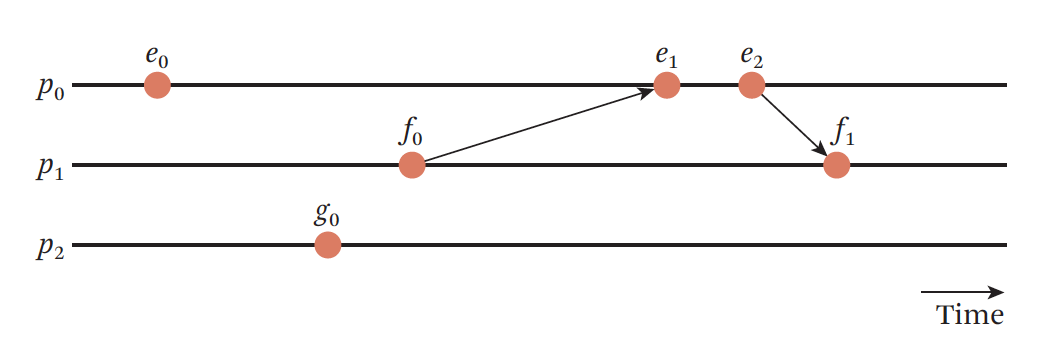Logical time

## Lamport timestamps: How they work

Lamport introduced the eponymous logical timestamps in 1978:

• Each individual process $$p$$ maintains a counter: $$LT(p)$$.
• When a process $$p$$ performs an action, it increments $$LT(p)$$.
• When a process $$p$$ sends a message, it includes $$LT(p)$$ in the message.
• When a process $$p$$ receives a message from a process $$q$$, that message includes the value of $$LT(q)$$; $$p$$ updates its $$LT(p)$$ to the $$\max(LT(p), LT(q))+1$$

For two events $$a$$ and $$b$$, if $$a \rightarrow b$$, then $$LT(a) < LT(b)$$.

Q: The reverse is not true: If $$LT(a) < LT(b)$$, then it does not mean that $$a \rightarrow b$$! Why?

## Why is the LT invariant not symmetric?

4 nodes exchange events.

Initial state of timestamps: $$[A(0), B(0), C(0), D(0)]$$

E1. $$A$$ sends to $$C$$: $$[A(1), B(0), C(0), D(0)]$$

E2. $$C$$ receives from $$A$$: $$[A(1), B(0), C(2), D(0)]$$

E3. $$C$$ sends to $$A$$: $$[A(1), B(0), C(3), D(0)]$$

E4. $$A$$ receives from $$C$$: $$[A(4), B(0), C(3), D(0)]$$

E5. $$B$$ sends to $$D$$: $$[A(4), B(1), C(3), D(0)]$$

E6. $$D$$ receives from $$B$$: $$[A(4), B(1), C(3), D(2)]$$

At this point, $$LT(E6) < LT(E4)$$, but it does not mean that $$E6 \rightarrow E4$$! Events 4 and 6 are independent.

## Vector clocks

Vector clocks can maintain causal order.

On a system with $$N$$ nodes, each node $$i$$ maintains a vector $$V_i$$ of size $$N$$.

• $$V_i[i]$$ is the number of events that occurred at node $$i$$
• $$V_i[j]$$ is the number of events that node $$i$$ knows occurred at node $$j$$

They are updated as follows:

• Local events increment $$V_i[i]$$
• When $$i$$ sends a message to $$j$$, it includes $$V_i$$
• When $$j$$ receives $$V_i$$, it updates all elements of $$V_j$$ to $$V_j[a] = \max(V_i[a], V_j[a])$$

## Vector clocks guarantees

• if $$a \rightarrow b$$, then $$VC(a) < VC(b)$$
• if $$VC(a) < VC(b)$$, then $$a \rightarrow b$$
• if $$VC(a) < VC(b)$$ and VC(b) < VC(c) then $$a \rightarrow c$$
• if $$VC(a) < VC(b)$$, then $$RT(a) < RT(b)$$, where RT is the clock time of events $$a$$ and $$b$$

Vector clocks are expensive to maintain: they require $$O(n)$$ timestamps to be exchanged with each communication.

However, it has been shown that we cannot do better than vector clocks!

# Distributed decision making

## What is true in a distributed setting?

• Nodes in distributed systems cannot know anything for sure
• Only make guesses
• Individual nodes cannot rely on their own information
• Clocks can be unsynchronized
• Other nodes may be unresponsive when updating state
• “Split-brain” scenarios: Parts of the system know a different version of the truth than the other part(s)

In distributed settings, the truth is determined by concensus, which is reached through voting mechanisms.

## Consensus

Making a decision in the presence of faulty nodes.

• Committing a transaction
• Synchronizing state machines## The 2 generals problemThe two generals problem setting

• 2 armies camped in opposing hills (A1 and A2)
• The are only able to communicate with messengers
• They need to decide on a time to attack
• Enemy (B) is camped between the two hills and can at any time intercept the messengers

Q How can the generals decide when to attack?

A It is impossible to make a reliable decision.

## The 2 generals problem solution

• The problem cannot be solved without loss of information
• Approximately:
• Pre-agree on timeouts
• Send $$n$$ labeled messages
• Receiver calculates received messages within time window, then decides how many messages to send for ack.

Consequences: we can only make distributed decisions using either reliable communication or more than 2 parties.

## The Byzantine generals problem

Formulated by Lamport et al., the Byzantine generals problem shaped distributed systems research for the next 40 years.

The formulation is simple:

• $$n$$ generals need to make unanimous decision
• they communicate with unreliable messages
• $$m$$ ($$n > m$$) generals vote suboptimally (i.e., lie) or do not vote